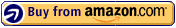In this workbook, children gain foundational skills for calculating fractions. Sections on greatest common factor and lowest common multiple prepare children for adding and subtracting fractions with unlike denominators, as well as multiplying and dividing fractions and mixed numbers.

Topics Covered in this Book:
-Reducing fractions to lowest possible denominator
-Factors and multiples
-Greatest common factor (GCF)
-Lowest common multiple (LCM)
-Addition & subtraction of fractions with unlike denominators
-Multiplication & division of fractions and mixed numbers
-Changing decimals into fractions
-Calculations with three fractions

8 1/2 x 11 inches. paperback. 96 pages. full color.

ISBN: 978-1-933241-60-9

#### US: \$7.95 | CA: \$8.95